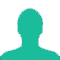# Out of 7 consonants and 4 vowels, how many words of 3 consonants and 2 vowels can be formed?

A. 210

B. 1050

C. 25200

D. 21400

E. None of these

### Solution(By Examveda Team)

Number of ways of selecting (3 consonants out of 7) and (2 vowels out of 4)
\eqalign{ & = \left( {{}^7{C_3} \times {}^4{C_2}} \right) \cr & = \left( {\frac{{7 \times 6 \times 5}}{{3 \times 2 \times 1}} \times \frac{{4 \times 3}}{{2 \times 1}}} \right) \cr & = 210 \cr}
Number of groups, each having 3 consonants and 2 vowels = 210
Each group contains 5 letters.
Number of ways of arranging 5 letters among themselves
= 5!
= 5 x 4 x 3 x 2 x 1
= 120
∴ Required number of ways = (210 x 120) = 25200

This Question Belongs to Arithmetic Ability >> Permutation And Combination

1.